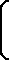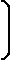Capt. Horatio T.P. Webb
Trigonometry Calculator for Right Triangles
Using HTML 5 Canvas
Parks -- Spring 2014
Version 1 -- Last Updated 6:00 PM 2/1/2014
Enter ANY two of the four
values shown below:
Canvas NOT supported by this browser
Enter Angle in degrees

OR
Use the slider below:
0°   90°
Hypotenuse
Side Opposite the Angle

Select Number of Digits to be
to the right of the Decimal
in calculations:

1. CASE 1: You know one angle and one distance.
A, B, C are angles and a ,b, and c are distances.

So, use one of these six formulae:

 sine (A°) = sin (A°) = oppositehypotenuse = ac cosecant (A°) = csc (A°) = 1sin(A°) = hypotenuseopposite = ca cosine (A°) = cos (A°) = adjacenthypotenuse = bc secant (A°) = sec (A°) = 1cos(A°) = hypotenuseadjacent = cb tangent (A°) = tan (A°) = oppositeadjacent = ab cotangent (A°) = cot (A°) = 1tan(A°) = adjacentopposite = ba

In this program we only use the first column above for calculations (sin, cos and tan). The second column formulae (csc, sec, and cot) are just the reciprocals of the first column values (i.e., csc(A°) = 1.0/sin(A°); sec(A°) = 1.0/cos(A°); and cot(A°) = 1.0/tan(A°) ).

2. CASE 2: You know two distance and NO angles.
Use these one of these three INVERSE formulae to find an angle:
A, B, C are angles and a ,b, and c are distances.
 A° = arcsinopposite hypotenuse= arcsina c“the set of all angles whose sin is the ratio of two sides    (the side opposite the angle and the hypotenuse)" A° = arccosadjacent hypotenuse= arccosb c“the set of all angles whose cos is the ratio of two sides    (the side adjacent to the angle and the hypotenuse)" A° = arctanopposite adjacent= arctana b“the set of all angles whose tan is the ratio of two sides    (the side opposite the angle and the side adjacent to the angle)"

In the range of angles 0° to 90° there is only one value for any arcsin, arccos, or arctan.